Courses

# Integer Type questions of Electrochemistry, Past year Questions JEE Advance, Class 12, Chemistry JEE Notes | EduRev

## Class 12 Chemistry 35 Years JEE Mains &Advance Past yr Paper

Created by: Abhishek Kapoor

## JEE : Integer Type questions of Electrochemistry, Past year Questions JEE Advance, Class 12, Chemistry JEE Notes | EduRev

The document Integer Type questions of Electrochemistry, Past year Questions JEE Advance, Class 12, Chemistry JEE Notes | EduRev is a part of the JEE Course Class 12 Chemistry 35 Years JEE Mains &Advance Past yr Paper.
All you need of JEE at this link: JEE

Integer Value Correct Type

1. All the energy released from the reaction X → Y, ΔrG° = –193 kJ mol–1 is used for oxidizing M+ as M+ → M3+ + 2e, E° = –0.25 V
Under standard conditions, the number of moles of M+ oxidized when one mole of X is converted to Y is [F = 96500 C mol–1] (JEE Adv. 2015)

Ans:  4

Solution :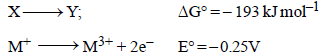Hence DG° for oxidation will be
ΔG° = – nFE°
= –2 × 96500 × (–0.25) = 48250 J = 48.25 kJ
48.25 kJ energy oxidises one mole M+

∴ 193 kJ energy oxidises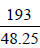mole M+ = 4 mole M+

2. The molar conductivity of a solution of a weak acid HX (0.01 M) is 10 times smaller than the molar conductivity of a solution of a weak acid HY (0.10 M). If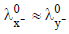the difference in their pKa values, pKa(HX) – pKa (HY), is (consider degree of  ionization of both acids to be <<1) (JEE Adv. 2015)

Ans:  3

Solution :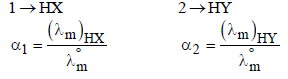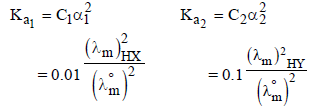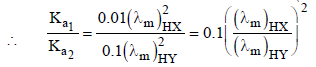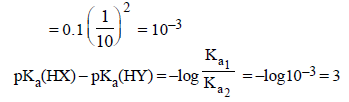Offer running on EduRev: Apply code STAYHOME200 to get INR 200 off on our premium plan EduRev Infinity!

69 docs|36 tests

,

,

,

,

,

,

,

,

,

,

,

,

,

,

,

,

,

,

,

,

,

,

,

,

,

,

,

,

,

,

;